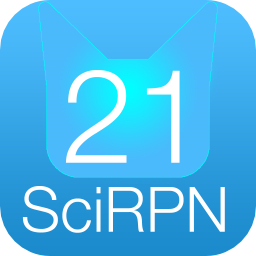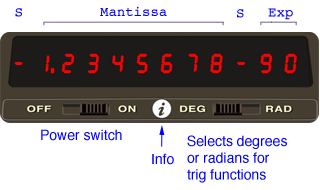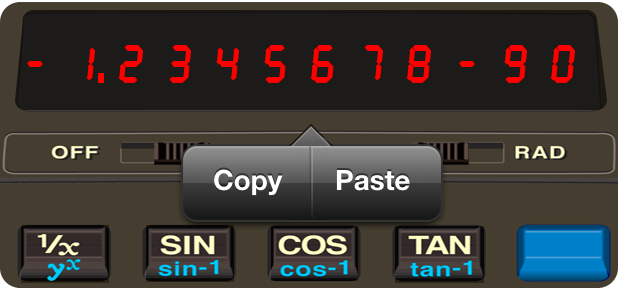GO-21 HelpGO-21
SciRPN
Pocket Calculator

GO-21 SciRPN closely simulates the venerable HP-21 Pocket Scientific Calculator. The HP-21 was released in 1975 and sold for a MSRP of \$125. Virtually indistinguishable from the real item in operation, GO-21 is a powerful yet simple retro calculator.

Capabilities At A Glance
• RPN (Reverse Polish Notation)
• Four stack registers
• One storage register
• Math, trigonometric, polar/rectangular, logarithmic, power functions
• Copy and paste
• Two separate windows for stack/storage registers and keystroke log
IntroductionThis document is not an exhaustive reference manual; rather, it's a terse outline of the calculator's function keys.

In this document normal calculator keys are shown in bold, such as +, or CHS, or 4, or SIN. Most keys can perform one other function, however, labeled in blue. To execute one of these functions you first touch the colored prefix key followed by the desired function key.So to compute the arc sine of a number you'd touch two keys, the blue prefix key followed by the SIN key. However, this document will simply show the key as sin-1: the font color implies the prefix character is used.

The Calculator Display

The GO-21 LED window acts as a display area, as well as a touch sensitive input area that initiates further activities not available on an actual HP-21.

The display shows the X stack register, usually the result of the latest calculation. Tapping the display brings up the Copy / Paste menu, allowing you to export the value of X, or import a new value of X.In GO-21, numbers in the display normally appear rounded to only two decimal places. For example, the fixed constant Π, which is actually in the calculator as 3.14159265358979, normally appears in the display as 3.14 (unless you tell the calculator to show you the number rounded to a greater or lesser number of decimal places).

Although a number is normally shown to only two decimal places, GO-21 always computes internally using each number as a 14-digit mantissa and a two-digit exponent of 10.

One or two keys control how numbers are displayed. DSP . (the DSP key followed by the decimal point key) allows numbers to be displayed in fixed decimal point format, and DSP alone displays numbers in scientific notation format. Display control alters only the manner in which a number is displayed, the actual number itself is not altered by any of the display control keys.

To select a display mode press one of the display modes followed by a number key to specify the number of decimal places (0-9) to which the display is to be rounded.

GO-21 extension: Engineering display mode is enabled using three keys, the blue shift key followed by DSP followed by a digit 1-5 (DSP n).

GO-21 switches the display from fixed point notation to full scientific notation (DSP 7) whenever the number is too large or too small to be seen with a fixed decimal point. This feature keeps you from missing unexpectedly large or small answers.

You can key in numbers multiplied by powers of 10 by pressing EEX (enter exponent of ten). You can save time when keying in exact powers of 10 by merely pressing EEX and then pressing the desired power of 10. To key in negative exponents of 10, key in the number, press EEX, press CHS to make the exponent negative, then key in the power of 10.

When a number is greater than 9.9999999 x 1099 an overflow has occurred and 9.9999999+99 is displayed instead. Other illegal operations, such as a divide by zero, display the word Error, then set the illegal number to zero.

The Stack

Automatic storage of intermediate results is the reason that GO-21 slides so easily through the most complex equations. The displayed X register, which is the only visible register in the calculator display, is one of four registers inside the calculator that are positioned to form the automatic memory stack. We label these registers X, Y, Z, and T. They are "stacked" one on top of the other with the displayed X register on the bottom.

The R↓ (roll down) and X⇔Y (X exchange Y) keys allow you to review the stack contents or to shift data within the stack for computation at any time. Each time you press the R↓ key the stack contents shift downward one register, with the contents of X rotating up to the T register. Notice that the contents of the registers are shifted - the registers themselves maintain their positions. Always remember, though, that it takes four presses of the R↓ key to return the contents to their original registers.

The X⇔Y (X exchange Y) key exchanges the contents of the X and Y registers without affecting the Z and T registers.

To clear the displayed X register only, press CLX. To clear the entire automatic memory stack, including the displayed X register, press CLR (clear stack). This replaces all numbers in the stack with zeros.

The ENTER key lifts the stack, by copying the contents of Z into T, Y into Z, X into Y, hence losing the contents of T and duplicating the contents of X.

The opposite of lifting the stack is called dropping the stack. When the stack drops, Y is copied to X, Z is copied to Y, and T is copied to Z, hence losing the contents of X and duplicating the contents of T.

Auxiliary Information Views

GO-21 provides views of the calculator's internal state unavailable on the real hardware. This makes using the calculator much easier. Access to this additional information is controlled by a swipe-up gesture on the calculator body proper, or a double-tap on the display. If the information view is visible, a swipe-up or double-tap hides the view. If the view is not visible, the information view appears. So a swipe-up or double-tap toggles the visibility of the auxiliary information.

The auxiliary view shows the 4 stack registers and the memory register. Also note in the lower-right corner a flip tab: touching the tab flips the window and shows up to 100 lines of key-press history.(Note: unlike for iPhone and iPod touch, which have tiny screens, on iPad the information view is by default always visible, but you can hide it with a swipe-up gesture or a double-tap, if desired.)

Function Keys
• Prefix Clear

To clear the blue prefix you have mistakenly pressed, merely press ENTER, then press the correct key.

• Reciprocals

To calculate the reciprocal of a number in the displayed X register, press 1/X.

• Square Roots

To calculate the square root of a number in the displayed X register, press √X.

• Using Pi

The value Π accurate to 14 places (3.14159265358979) is provided as a fixed constant in GO-21. Merely press Π whenever you need it in a calculation.

• Storage Register

In addition to automatic storage of intermediate results that is provided by the four-register automatic memory stack, GO-21 also has one addressable storage register that is unaffected by operations within the stack. This storage register allows you to set aside a number as a constant or for use in later calculations.

To store a value appearing in the display, press STO. When a number is stored in memory, it is merely copied into the storage register.

To copy a number from the storage register into the displayed X register, press the RCL key.

Recalling a number causes the stack to lift unless the preceding keystroke was ENTER or CLX.

When you recall a number, it is copied from the storage register into X, and it also remains in the storage register. You can recall a number from the storage register any number of times without altering it; the number will remain in the storage register as a 10-digit number with a two-digit exponent of 10 until you overwrite it by storing another number there, or until you clear the storage register.

To clear the number from the storage register, simply store the quantity zero in the register by pressing 0 STO.

• Storage Register Arithmetic

Arithmetic is performed upon the contents of the storage register by pressing M+, M-, Mx or .

• M+ (X added to storage register, sum placed in storage register.)
• M- (X subtracted from storage register, difference placed in storage register.)
• Mx (X multiplied by storage register, product placed in storage register.)
• (Storage register divided by X, quotient placed in storage register.)

When storage register arithmetic operations are performed, the answer is written into the storage register, while the contents of the displayed X register and the rest of the stack remain unchanged.

If the magnitude of a number in the storage register exceeds 9.999999999 x 1099, the display immediately shows OF (overflow) to indicate that a storage register has overflowed.

• Trigonometric Functions

GO-21 provides you with six trigonometric functions. It also calculates angles in decimal degrees or radians. Use the slide switch to select DEG or RAD.

The six trigonometric functions provided by the calculator are:

• sin (sine)
• sin-1 (arc sine)
• cos (cosine)
• cos-1 (arc cosine)
• tan (tangent)
• tan-1 (arc tangent)

Each trigonometric function assumes angles in decimal degrees or radians. Trigonometric functions are one-number functions, so to use them you key in the number, then press the function keys.

• Polar/Rectangular Coordinate Conversion

Two functions are provided for polar/rectangular coordinate conversion. To convert values in the X and Y registers, (representing rectangular x, y coordinates, respectively) to polar r, Θ coordinates (magnitude and angle, respectively), press →P. Magnitude r then appears in the X register and angle Θ is placed in the Y register.

Conversely, to convert values in the X and Y registers (representing polar r, Θ respectively) to rectangular coordinates (x, y respectively), press →R. Coordinate x then appears in the X register and coordinate y is placed in the Y register.

• Logarithms

GO-21 computes both natural and common logarithms as well as their inverse functions (antilogarithms):

• ln is loge (natural log). It takes the log of the value in X to base e (2.718...).
• ex is antiloge (natural antilog). It raises e (2.718...) to the power of the value in X. (To display the value of e, press 1 ex.)
• log is log10 (common log). It computes the log of the value in the X register to base 10.
• 10x is antilog10 (common antilog). It raises 10 to the power of the value in the X register.

• Raising Numbers to Powers

Yx permits you to raise a positive number (either an integer or a decimal) to any power. In conjunction with 1/X, Yx provides a simple way to extract roots. For example, the cube root of 5 is equivalent to 51/3.

Improper Mathematical Operations

If you attempt a calculation containing an improper operation - say, division by zero - the display will show Error. To clear, press CLX.

The following are improper operations:

• ÷ where X = 0
• Yx where Y < 0 with X not an integer, or where Y = 0 with X < 0
• √X where X < 0
• 1/X where X = 0
• log where X <= 0
• ln where X <= 0
• sin-1 where absolute value of X > 1
• cos-1 where absolute value of X > 1
• where X = 0
Gesture Summary
• Shake to clear X.
• Single tap the display for Copy / Paste.
• Swipe-up to show/hide the stack/memory view.
• Double tap the display to show/hide the stack/memory view.
• Two-finger pan for iPad to reposition the stack/memory and calculator views.
• Tap blue-hatched flip tab to alternate between stack/memory and paper tape views.
• Swipe left and right to manipulate slide switches, if activated in Settings.
• Flick left on the display to erase last mantissa character entered.
• Triple tap the display to show/hide the Info button.